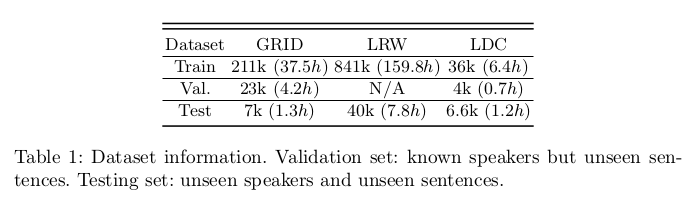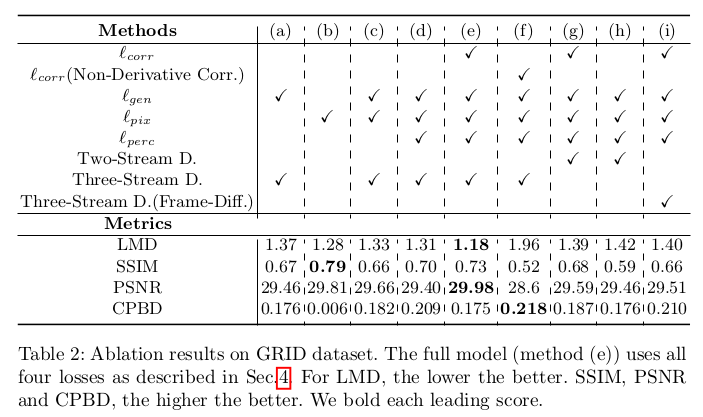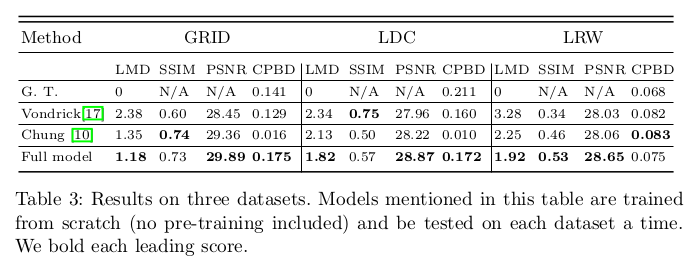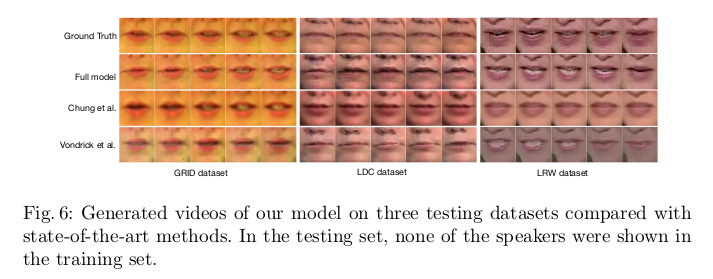# 论文引入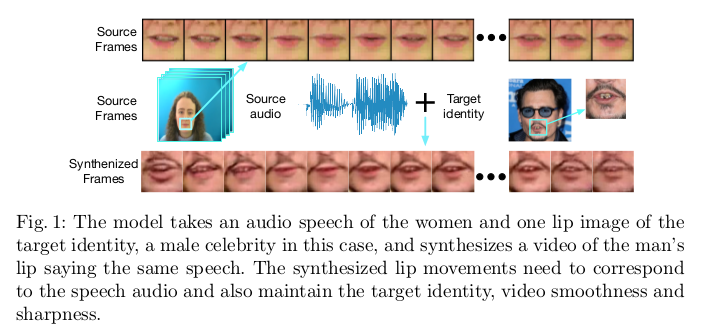• 第一个考虑语音和唇部运动之间的相关性，一目了然地生成多个唇部图像
• 构建和训练唇部运动发生器网络时探索各种模型和损失函数
• 量化了评估指标，对训练集外的唇部图像依然适用

# 模型结构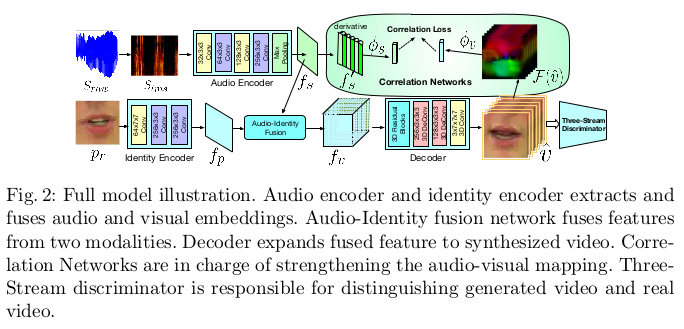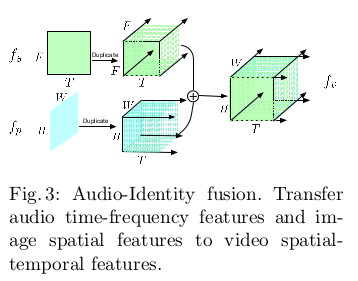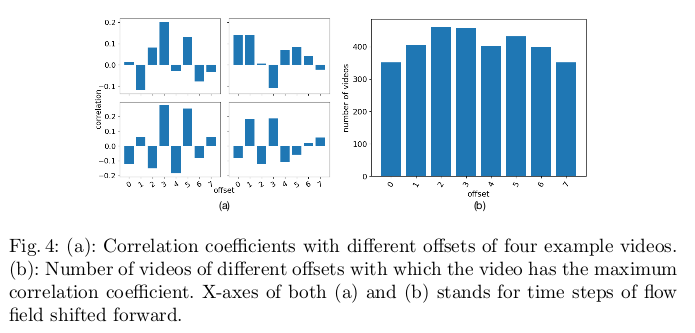$\mathcal L_{corr} = 1 - \frac{\phi_s(f_s^,) \cdot \phi_v(\mathcal F(v))}{\Vert \phi_s(f_s^,) \Vert_2 \cdot \Vert \phi_v(\mathcal F(v)) \Vert_2}$

$\mathcal L_{pix}(\hat{v},v) = \Vert v - \hat{v} \Vert$

$\mathcal L_{perc}(\hat{v},v) = \Vert \varphi (v) - \varphi (\hat{v}) \Vert_2^2$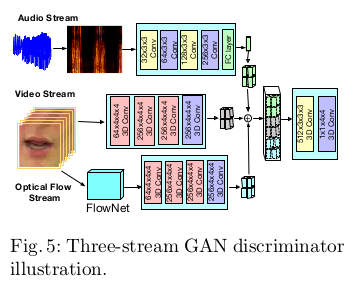$\mathcal L_{dis} = -log D([s^j,v^j]) - \lambda_p log(1 - D([s^j,\hat{v}])) - \lambda_u log(1 - D([s^j,v^k])), k \neq j$

$\mathcal L = \mathcal L_{corr} + \lambda_1 \mathcal L_{pix} + \lambda_2 \mathcal L_{perc} + \lambda_3 \mathcal L_{gen}$

# 实验